WHCSRL 技术网

# [C语言] 函数：库函数--自定义函数--函数的调用--练习--详解＜个人＞------（1）

• 前言
• 一. 函数是什么？
• 二. C语言中函数的分类
• 三. 库函数
• 四. 自定义函数
• 五. 函数的调用
• 六. 练习
• 总结

## 前言

[ C语言 ]循环语句---while---for---do while---详解及练习题巩固_小白又菜的博客-CSDN博客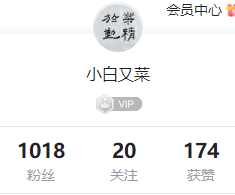其实我开始写博客的初衷是记录自己的学习过程，通过写博客的方式对知识进行再次总结，加深自己对知识的记忆，我现在的目的如此，以后仍然是！每每看到关注量，浏览量这些数据增加，都会让我变得开心，这是大家给予我的一份肯定。在此，感谢各位一直以来的支持，我以后也会对自己的知识记录更加详细，更加丰富，在记录我进步的同时，希望大家通过看到我的博客也会进步。我们一起朝着自己的大厂梦前进吧！！！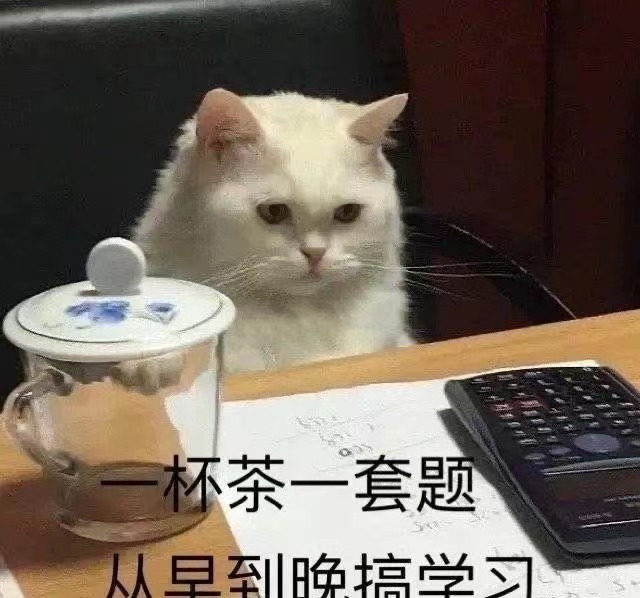话不多说，上车！！！

———————————————————————————————————————————                                                            ****正文开始****

## 一. 函数是什么？

subprogram, callable unit），是一个大型程序中的某部分代码， 由一个或多个语句块组

1.   库函数
2.   自定义函数

## 三. 库函数

Q : 为什么会有库函数？

A ：

1. 我们知道在我们学习C语言编程的时候，总是在一个代码编写完成之后迫不及待的想知道结果，想把这个结果打印到我们的屏幕上看看。这个时候我们会频繁的使用一个功能：将信息按照一定的格式打印到屏幕上（printf）。

2. 在编程的过程中我们会频繁的做一些字符串的拷贝工作（strcpy）。

3. 在编程是我们也计算，总是会计算nk次方这样的运算（pow）。

## i>举例：strlen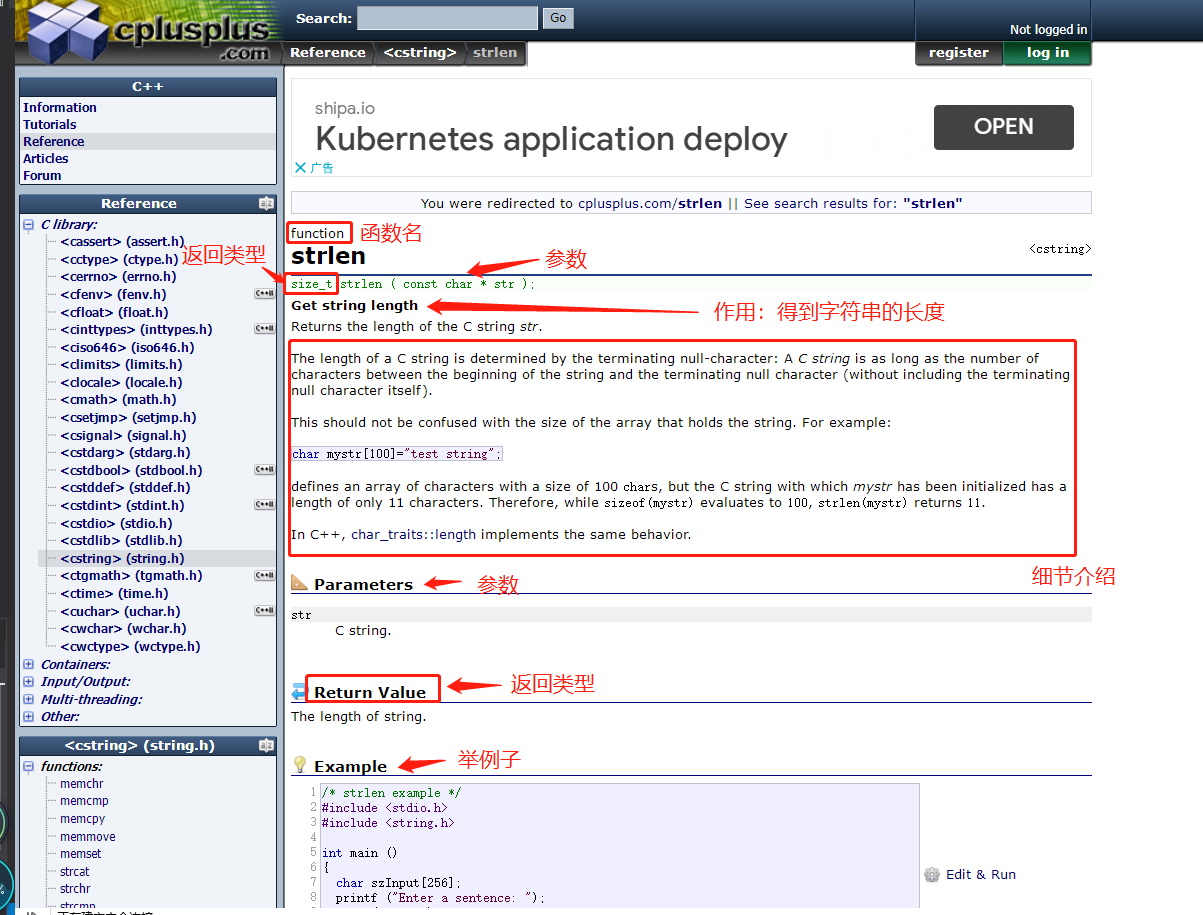``````#include <stdio.h>#include <string.h>int main() {	char arr[] = "abc";	// size_t  -> unsigned int	size_t len = strlen(arr);	printf("%%u
", len);	// %%d  - 有符号	// %%u  - 无符号 （没有负数）	return 0;}``````

##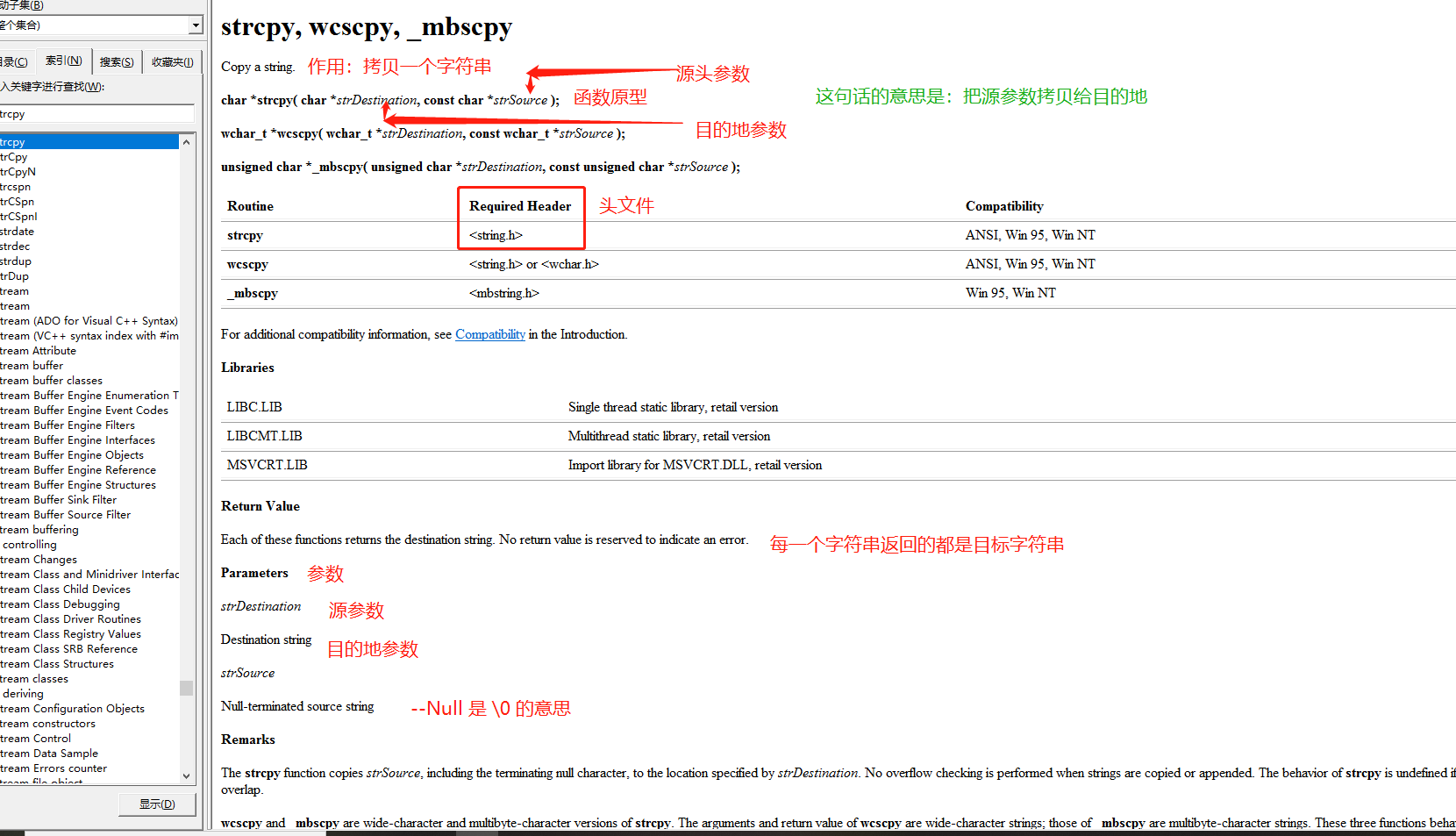``````#include <stdio.h>#include <string.h>int main() {	char arr1 = { 0 };//  目的地	char arr2[] = "hello" ;// 源数据	strcpy(arr1, arr2);	printf("%%s
",arr1); 	return 0;}``````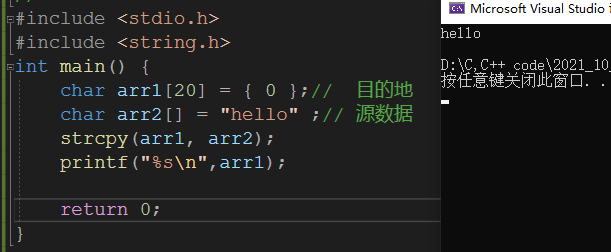## iii>举例：memset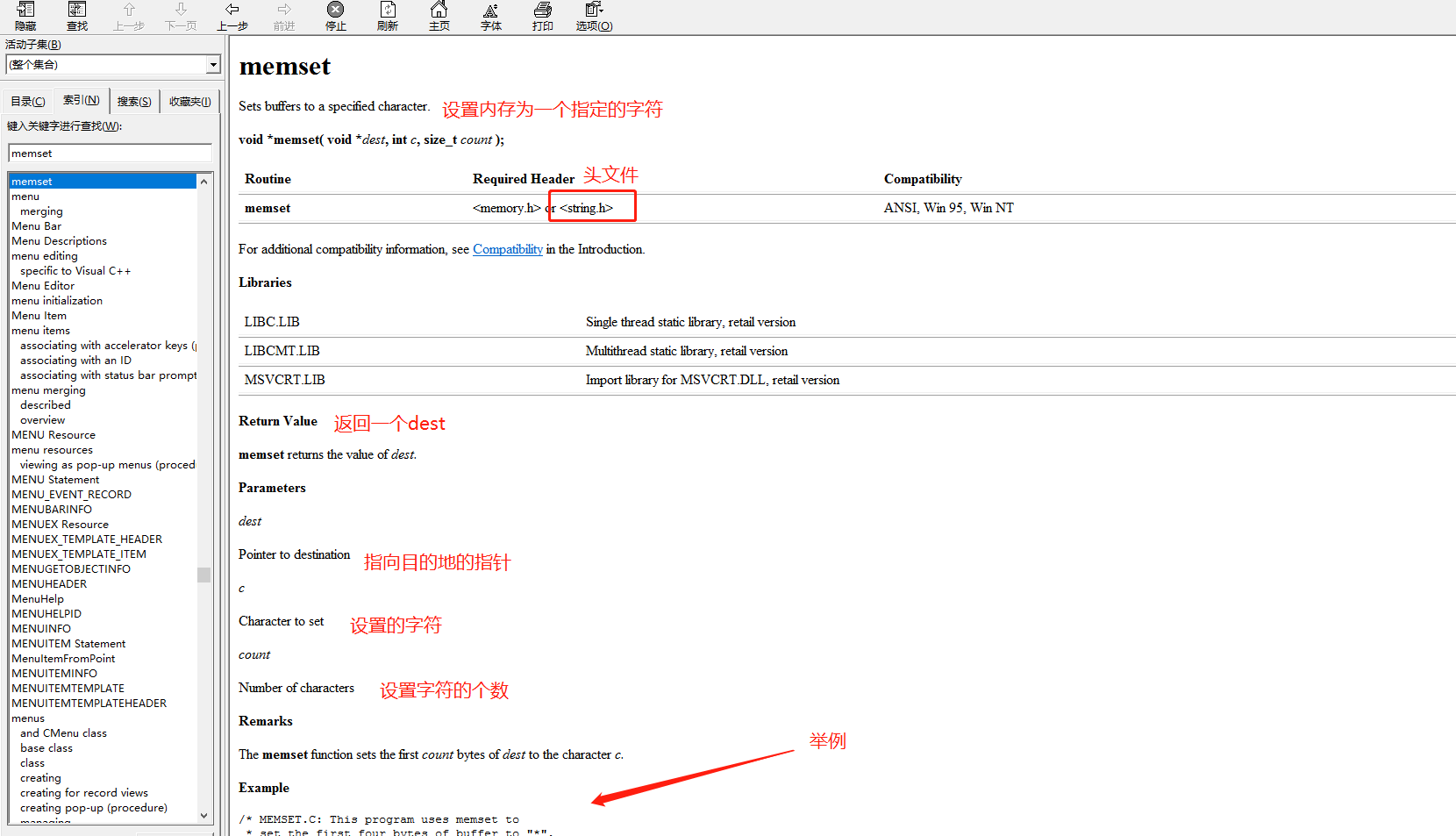``````#include <stdio.h>#include <string.h>int main(){	char arr[] = "hello world";	memset(arr, 'x', 5);	printf("%%s
", arr); 	return 0; }``````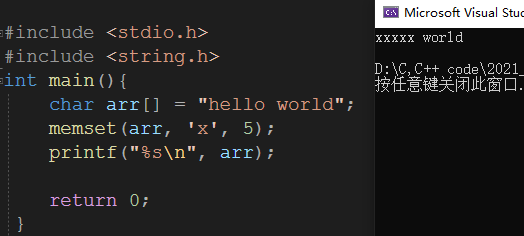IO函数

## 2>函数的组成

``//***自定义函数***// 函数返回类型  函数名   参数// ret_type fun_name(para1,*) // {                             //     //   statement; // 语句项         //函数体//               }               //ret_type 返回类型fun_name 函数名para1    函数参数``

## 3>举例

### 一：写一个函数可以找出两个整数中的最大值。

``````// 写一个函数，找出两个数的最大值//返回类型   函数名   参数int get_max(int x, int y) {	int z = 0;	z = (x > y ? x : y);	return z;} int main() {	int a = 0;	int b = 0;	scanf("%%d %%d", &a, &b);	int m = get_max(a, b);	printf("%%d
", m); 	return 0;}``````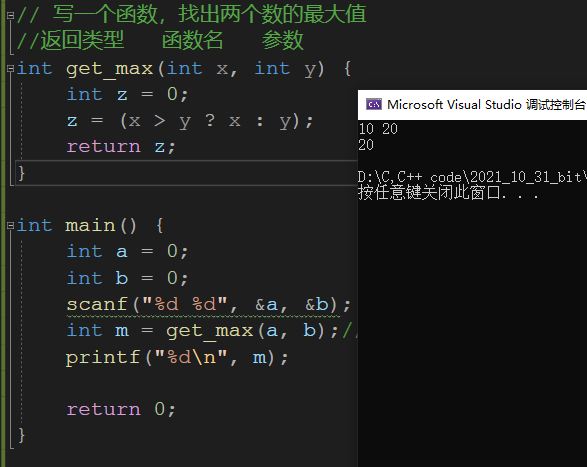### 二：写一个函数可以交换两个整形变量的内容。

``````void Swap1(int x, int y) {	int  temp = 0;	temp = x;	x    = y;	y    = temp;}int main() {	int a = 0, b = 0;	scanf("%%d %%d", &a, &b); 	// 交换两个变量	printf("交换前a = %%d, b = %%d
", a, b);	Swap1(a,b);   // 传值调用	printf("交换后a = %%d, b = %%d
", a, b); 	return 0;}``````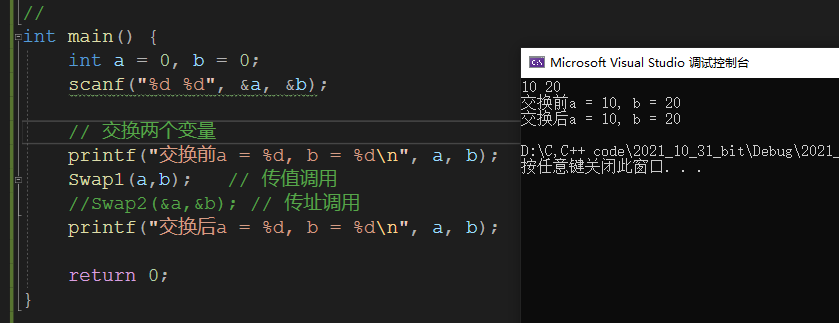`````` 正确写法    使用指针void Swap2(int* pa,int* pb) {	int t = 0;	    t = *pa;	  *pa = *pb;	  *pb = t; }int main() {	int a = 0, b = 0;	scanf("%%d %%d", &a, &b); 	// 交换两个变量	printf("交换前a = %%d, b = %%d
", a, b);	Swap2(&a,&b); // 传址调用	printf("交换后a = %%d, b = %%d
", a, b); 	return 0;}``````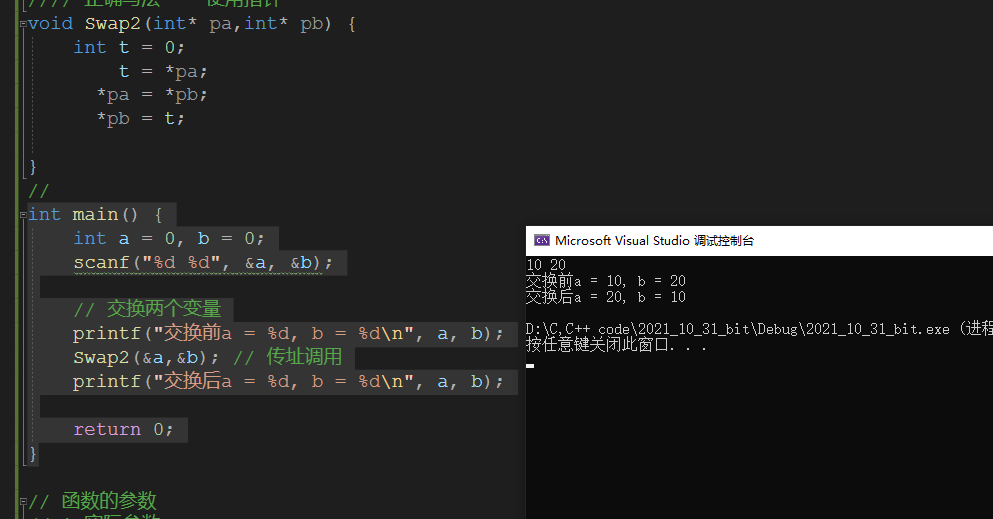##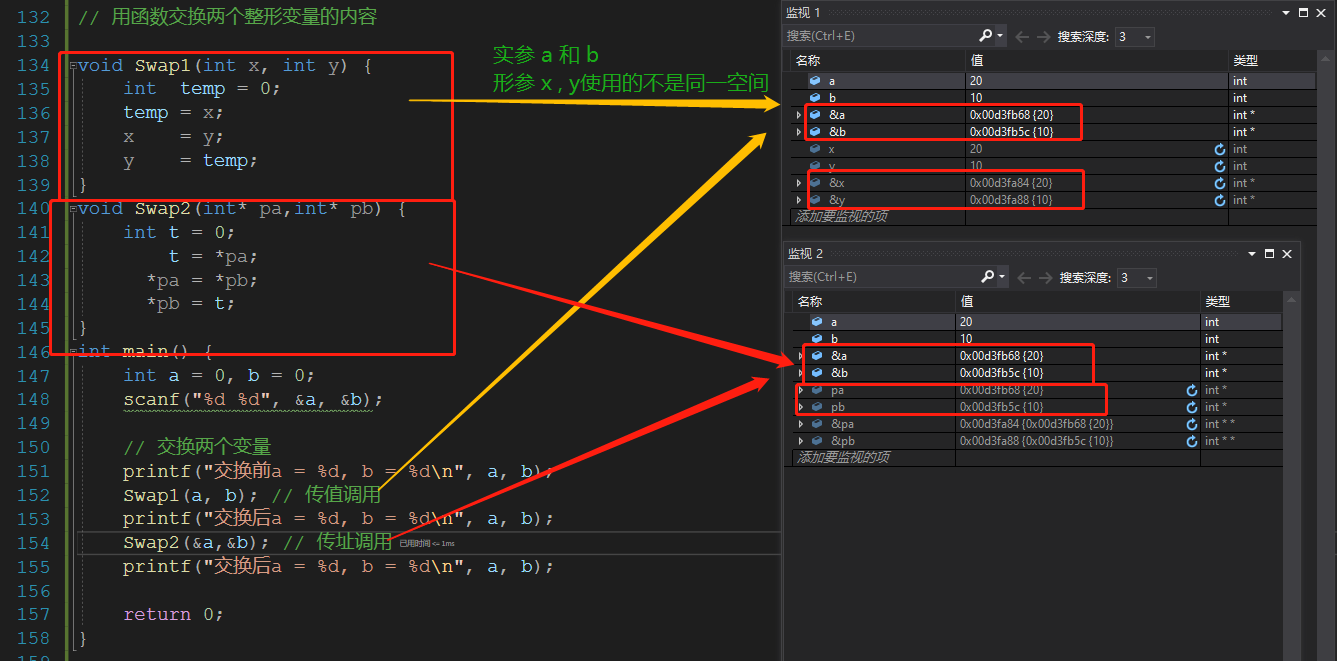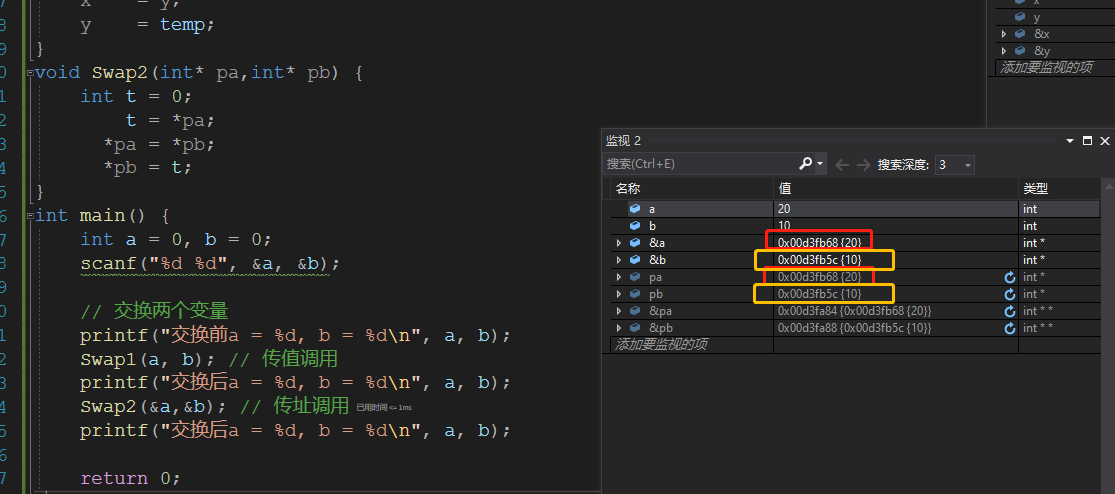## 六. 练习

`` //is_prime() //是素数返回 1 ，不是素数返回 0int is_prime(int n) { 	// 2 ~ n-1 的数字试除	int j = 0;	for (j = 2; j <= sqrt(n); j++) {		if (n %% j == 0) {			return 0;		}			}	return 1; } int main() {	int i = 0;	for (i = 100; i <= 200; i++) { 		// 判断 i 是否为素数 - 如果是素数就打印		// i 取 100 - 200		if (is_prime(i) == 1) {			printf("%%d ", i);		}	} 	return 0;}``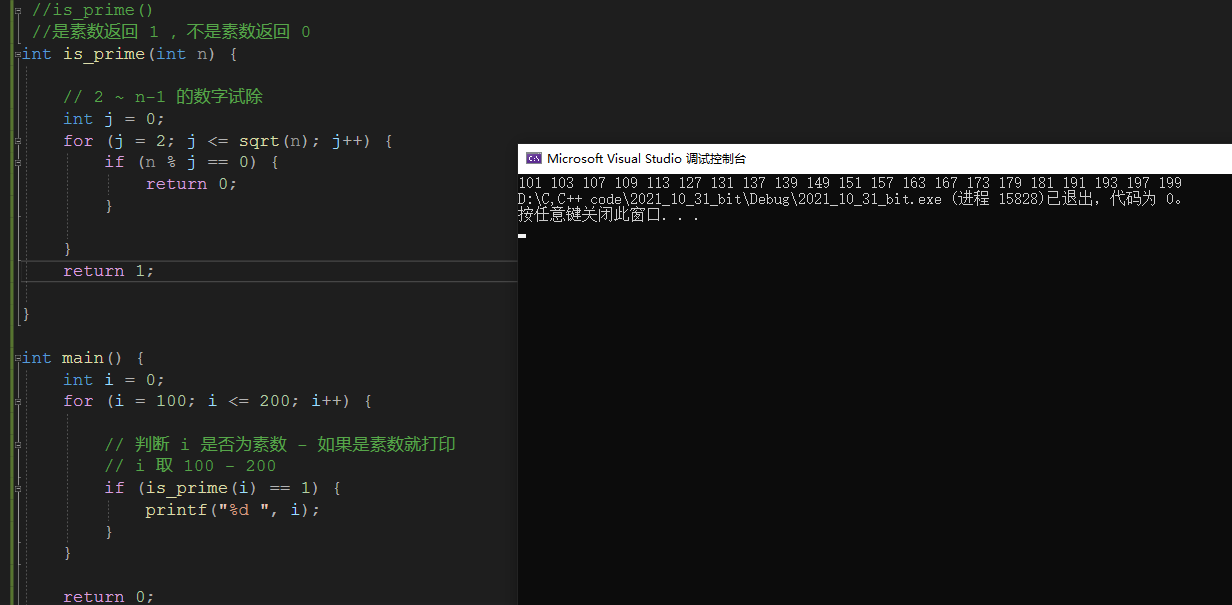`` //写一个函数判断一年是不是闰年 //是闰年 返回1 不是闰年 返回0int is_leap_year(int y) {	//if (y %% 100 != 0 && y %% 4 == 0 || y %% 400 == 0) {	//	return 1;	//}	//else {	//	return 0;	//} 	return (y %% 100 != 0 && y %% 4 == 0 || y %% 400 == 0);}int main() { 	int y = 0;	for (y = 1000; y <= 2000; y++) { 		// 判断 y 是不是闰年		if (is_leap_year(y) == 1) {			printf("%%d ",y);		}	} 	return 0;}``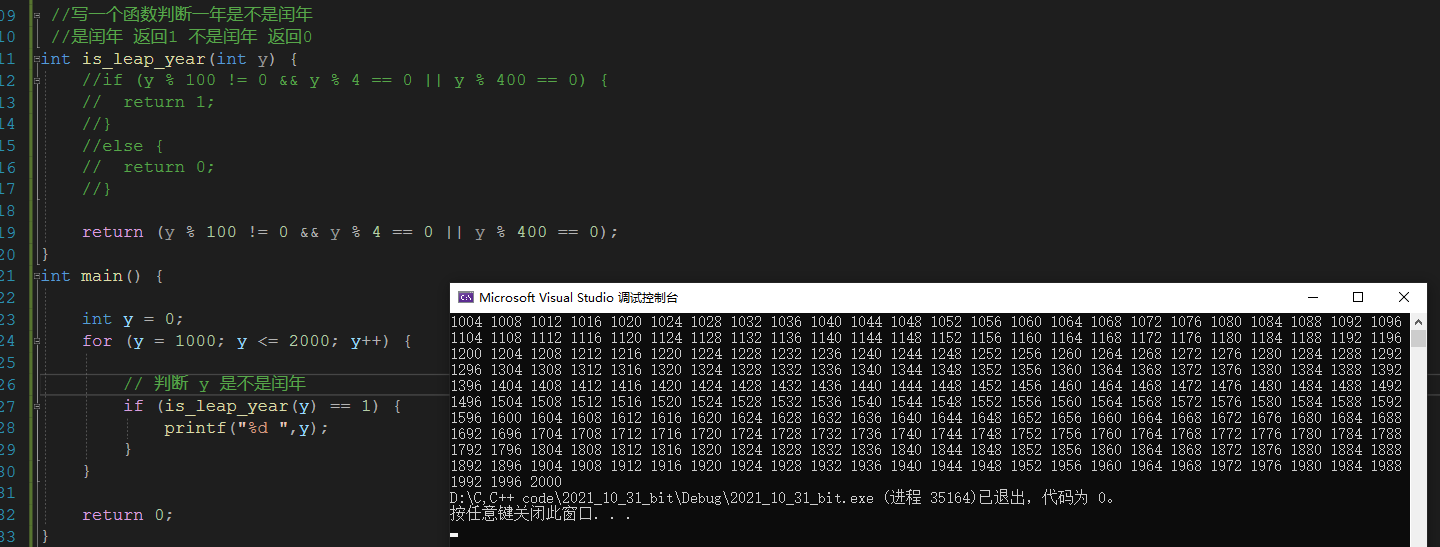``````//写一个函数 ，实现一个整形有序数组的二分查找//如果找到了返回 下标//如果找不到返回  -1 int binary_search(int arr[], int k,int sz) {	int left = 0;	int right = sz - 1; 	while (left<=right) {		int mid = (left + right) / 2;		if (arr[mid] < k) {			left = mid + 1;		}		else if (arr[mid] > k)		{			right = mid - 1; 		}		else if (left == right) {			return mid;		}	}	return -1; }int main() {	int arr[] = { 1,2,3,4,5,6,7,8,9,10 };	int k = 7;	int sz = sizeof(arr) / sizeof arr;	   //      40        /  4	// 数组arr传给binary_search函数的时候，其实传递的arr数组首元素的地址	int ret = binary_search(arr,k,sz); // 在arr数组中找k	if (-1 == ret) { 		printf("找不到
");	}	else {		printf("找到了，下标是%%d
", ret);	}	return 0;}``````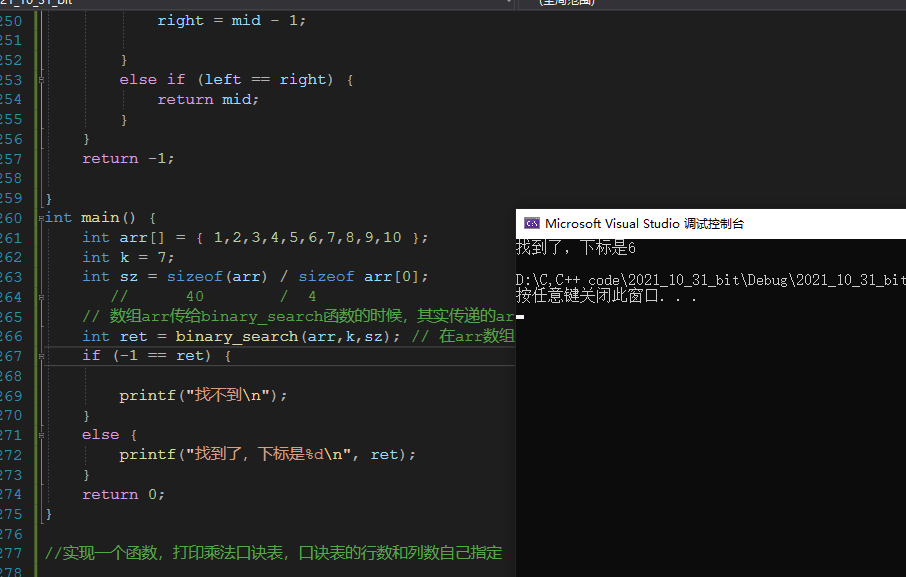``````void Multiplication_formula_table(int i, int j,int n) {	for (i = 1; i <= n; i++) {		for (j = 1; j <= i; j++) {			printf("%%d*%%d=%%d ", j, i, i* j);		}		printf("
");	}}int main() {	int n = 0;	scanf("%%d",&n);	int i = 0;	int j = 0;	Multiplication_formula_table(i ,j,n);	return 0;}``````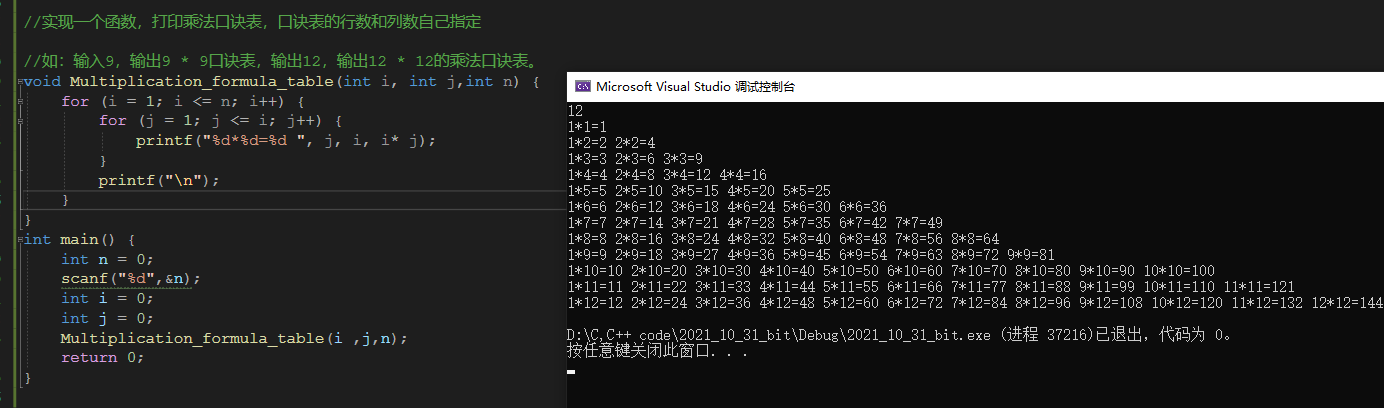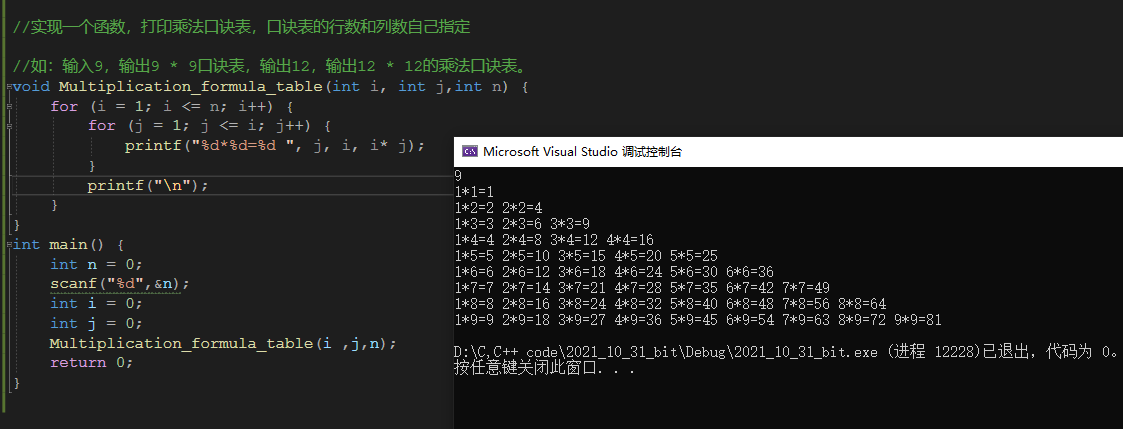****正文结束****

——————————————————————————————————————————